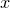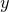## 8.2.5 Steps

The following plot styles allow data to be plotted with a series of horizontal steps associated with each supplied data point:

• steps

• fsteps

• histeps

Each of these styles takes two columns of data, containing the- and-coordinates of each data point. They expect identically-formatted data regardless of whether they are used on two- and three-dimensional plots; in the latter case, the steps are drawn in the plane.

An example of their appearance is shown in Figures 8.2(d), (e) and (f); for clarity, the positions of each of the supplied data points are marked by red crosses.

These plot styles differ in their placement of the edges of each of the horizontal steps. The steps plot style places the right-most edge of each step on the data point it represents. The fsteps plot style places the left-most edge of each step on the data point it represents. The histeps plot style centers each step on the data point it represents.